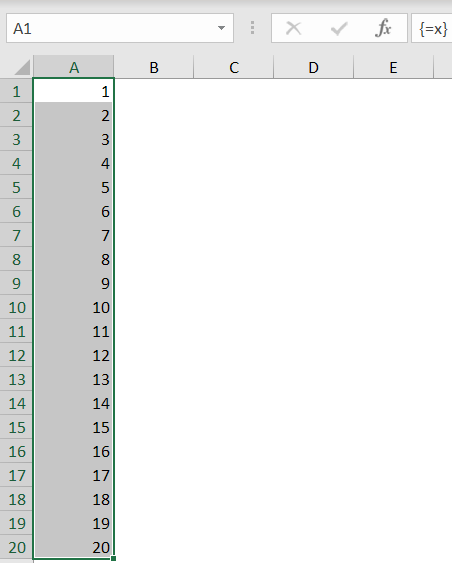## Subscribe to our mailing list

* indicates required

Audit !!!

Check out our RefTreeAnalyser
the ultimate Excel formula auditing tool.

Trainings

Excel VBA Masterclass (English)
Excel VBA for Financials (Dutch)

Third party tools

FastExcel
The best tool to optimise your Excel model!

Stellar Phoenix Excel Repair
Best tool to repair corrupt Excel sheets and objects
Home > English site > Articles > Chart an Equation > x-values# Charting a mathematical equation using Excel and defined names

## Getting x values

The next task is to get a list if x values we can use for the x-axes of the chart. A little known fact, is that when a defined name is used to name a formula this formula will be an array formula by default. We're going to put that to use.

First we need a set of incrementing numbers. We'll use the ROW worksheet function for that.

When we define this name local to worksheet Sheet1:

Name: Sheet1!x

RefersTo: =ROW(1:20)

and we enter an array formula into cells A1:A20 (select A1:A20 in Sheet1 and type =x, then hit control-shift-enter) we get this result:But we want the number of x-values to be dependent on a number entered into a cell. We'll use the OFFSET worksheet function for this purpose:

=ROW(OFFSET(Sheet1!\$A\$1,0,0,20,1))

If we replace the previous formula in "Sheet1!x" with the one above, the result will remain the same as shown above.
All it takes now is replace the fixed 20 with a cell reference:

=ROW(OFFSET(Sheet1!\$A\$1,0,0,Sheet1!\$B\$5,1))

Now we have the numbers 1 to 20 (or up to whatever number we enter into cell B5 on sheet1).

But we need more flexibility, we want to be able to set a minimum and a maximum value for x and use the 1-20 range to space out these limits.

Let's define these named ranges:

 Name Refers To xStart =Sheet1!\$B\$3 xEnd =Sheet1!\$B\$4 xNumberOfPoints =Sheet1!\$B\$5 xRange =xEnd-xStart

To get a series of "xNumberOfPoints" from xStart to xEnd, the following formula applies:

xPoint=xStart+xRange/(xNumberOfPoints-1)*Counter(1 to xNumberOfPoints-1)

So applying the approach depicted above:

=xStart+xRange/(xNumberOfPoints-1)*(ROW(OFFSET(Sheet1!\$A\$1,0,0,xNumberOfPoints,1))-1)

We'll name this new formula (surprisingly): Sheet1!x

### Note:

Editing and creating names is much easier with the free Name Manager.